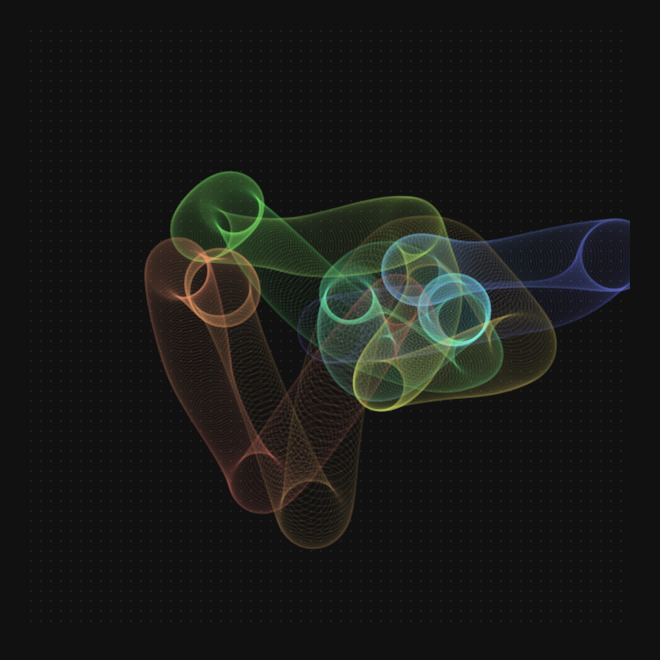## Ronin experiment 11 - traces of a restless ghost

I worte a ronin script that traces the paths of a restless ghost trying to figure a way out of the screenThis is the ghost's source code

``````(resize 600 600)
(clear)
(defn hsla (h s l a) (
(concat "hsla(" h "," s "%," l "%," a ")" )
))

(def p { :x 300 :y 300 })
(def s { :x 0 :y 0 })
(def a { :x 0.9 :y -0.1 })
(def f { :x 0 :y 0 })
(def damp 0.92)

(defn update () (

(set p "x" (add p:x s:x))
(set p "y" (add p:y s:y))

(set s "x" (mul damp (add s:x a:x)))
(set s "y" (mul damp (add s:y a:y)))
(set a "x" (mul damp (add a:x f:x)))
(set a "y" (mul damp (add a:y f:y)))

(set f "x" (mul damp f:x))
(set f "y" (mul damp f:y))

(if (eq (floor (random 30)) 1) (
(if (gt p:x 400)
(set f "x" (add f:x (random -0.1 0.05)))
(set f "x" (add f:x (random -0.05 0.1)))
)
(if (gt p:y 300)
(set f "y" (add f:y (random -0.1 0.05)))
(set f "y" (add f:y (random -0.05 0.1)))
)
))

(if (gt p:x frame-rect:w) (
(set p "x" frame-rect:w)
(set s "x" (mul -1 s:x))
))
(if (lt p:x 0) (
(set p "x" 0)
(set s "x" (mul -1 s:x))
))
(if (gt p:y frame-rect:h) (
(set p "y" frame-rect:h)
(set s "y" (mul -1 s:y))
))
(if (lt p:y 0) (
(set p "y" 0)
(set s "y" (mul -1 s:y))
))
))

(defn draw () (
;(fill (frame) "#00000002")
(stroke
(circle p:x p:y (add 30 (mul 6 (sin (time 0.004)))))
2 (hsla (add 180 (mul 180 (sin (time 0.000075)))) 80 70 0.05))
(update)
))

(on "animate" draw)
``````